# EIP 1559: A transaction fee market proposal¶

## TL;DR¶

• EIP 1559 is a proposed improvement for the transaction fee market. It sets a variable "base" gasprice to be paid by the user and burned by the protocol, in addition to a "tip" paid by the user to the block producer.
• When many transactions compete for limited space, the basefee goes up. When few transactions are included in the block, the basefee goes down.
• We simulate simple demand scenarios and progressively iterate, painting a picture of the mechanism's dynamics. We leave analysis of strategic interactions and a more sophisticated demand behaviour for later work.

EIP 1559 is perhaps one of the most anticipated upgrades to the economic model of Ethereum. Proposed by Vitalik Buterin in his Blockchain Resource Pricing paper, the mechanism seeks to replace the first-price auction model governing the current fee market for transaction inclusion.

## Why EIP 1559?¶

### Transactions 101¶

Users submit transactions to interact with a blockchain. In Ethereum, these transactions can be as simple as sending ether from one account to another, or as complex as making a contract perform something on your behalf (entering a lottery, executing a trade etc). In the latter case, your transaction might trigger other transactions to execute, cascading until no more side-effects take place. It is clear that the simple transfer is much less computation than a complex, multistage transaction, so we measure how much resources each transaction requires by how much gas the transaction (and its potential cascading transactions) needs to execute.

The Ethereum Virtual Machine (EVM), which processes these transactions, has a cost in gas for each elementary operation: adding numbers, storing a value, transfering ether. All transactions are made up of an arbitrary number of these elementary operations (even the really complex ones!) Together, these elementary operations tally up to the gas necessary to process the entire transaction.

Transaction operations Gas
2 Transfer 100
Transaction total 120

But space is scarce, as blocks are produced on a regular interval and offer only a limited amount of available gas. To avoid congestion, transaction senders ("users") price the gas they seek to use, e.g., specifying that they are ready to pay 6 Gwei per unit of gas to have their transaction included.

Transaction gas Gas price (Gwei) Total fee (Gwei)
120 6 720

### Auctions as market mechanism¶

In the current auction model, block producers typically select a set of the highest paying transactions, making sure that the total gas required by all transactions in the set does not exceed the limit amount of gas offered in a block. Users with selected transactions end up paying the gas price they specified, times the amount of gas their transaction required to be processed. Thus, users "pay what they bid": this is a first-price auction.

In the following table, we assume the block gas limit is 1,000. Though there is a transaction (the last one) paying out 700 Gwei, the block producer cannot include it as it would break the gas limit and thus prefers to include the first, 720 Gwei-valued, transactions. Users pay exactly the fee stated in the column.

Transaction gas Gas price (Gwei) Total fee (Gwei) Included? Cumulative gas
120 6 720 Yes 120
200 5 1000 Yes 320
700 1 700 No 320

The first-price auction has many defects. In its simplest theoretical model, where users bid simultaneously and privately, it is a well-known result that first-price auction are not incentive-compatible, meaning that users do not have the incentive to bid their true value for the transaction being included (you can see why in these slides by Jackson, Leyton-Brown and Shoham, from slide 5 onwards). Second-price auctions are though, when the winner of the auction pays the bid offered by the second-highest bidder.

So second-price auctions were considered as an alternative, but while we could hold Sotheby's accountable for manipulating the result of an auction, we can't quite always do that in the blockchain setting. Since block producers have the final say on who gets included or not, they can "stuff" the blocks with phony transactions from themselves to pump up the "second price", and thus trick the protocol into giving them more than what the market should offer while leaving honest fee-paying transactions by the curb (this can be somewhat mitigated (Basu et al., 2019) (and there are even results (Akbarpour, Li, 2019) for the case when Sotheby's cannot be held accountable)).

### Pricing congestion and EIP 1559¶

Enters EIP 1559, written up in an Ethereum improvement protocol (EIP) suggestion by Eric Conner of EthHub fame and Buterin himself. The proposal involves setting a variable basefee to be paid by anyone who wishes to have a transaction included, with the base fee varying according to how empty or how full blocks are. The block limit is set to a fairly high value, while the EIP 1559 mechanism targets a fixed block size (the amount of gas spent by transactions in the block).

In dire times, when everyone is trying to upgrade their CryptoKitty or frantically closing out their debt positions, block sizes increase as more users are willing to pay the current basefee. In this case, the mechanism increases the basefee to price out users who just don't want it bad enough, returning block sizes to their fixed target. Alternatively, when the chain is an open empty road to nowhere, we would prefer to encourage users to send their transactions in for cheap, so basefee should be much lower.

Under the auction market though, if a lot of people want to transact on the chain at the same time, the threshold bids making it into the blocks should steadily increase (and indeed, have done so in the past). Isn't that the same outcome as EIP 1559? Complicating things, we'll also see in our simulations that users can specify a "tip" paid out to the block producers, in addition to the basefee. A higher tip gets you to the end of the line faster, kind of like the auction mechanism does already. So why do we care about a mechanism seemingly not so different from the auction?

Basefee (burned) Tip (paid to block producer) Total fee
4 1 5

One clue is predictability. First-price auctions are notoriously hard to analyse even in the best of cases. It gets worse when bids are open (as they are to anyone who listens to the mempool where user transactions languish before being included) and replaceable (as they are, though not so trivially, with transaction-replacement operations). There is hope that a more "algorithmic" price discovery mechanism will help remove this variability, even when demand fluctuates swiftly (we'll see some of that in the simulations below).

Another clue is analytic simplicity. Pricing things, and especially congestion, in the most parsimonious manner is one object of algorithmic game theory, which is the study of mechanisms from the computational lens. We know from Pigou, Vickrey and a long line of economists that we usually like to internalise externalities, i.e., make people pay for not only what they are doing for themselves, but also what they are doing to others.

When I am on the road in the morning, I impose my presence to everyone else also on the road, and vice-versa. This is why the most fundamental result in this pillar of the discipline states that the correct price to pay for people cramming in some resource is exactly the marginal price: the price of the extra inconvenience for everyone else that your presence imposes. With first-price auctions, it is not clear that we ever get to that price. But a mechanism seeking to achieve some target (e.g., the amount of gas used by a block) and raising/lowering the price (e.g., the basefee) to meet that target gets much closer to this marginal price. And if that is simpler for us to analyse, that also means we get to predict a bit better how the mechanism will behave. Double whammy!

But ok, after this long intro, let's dive into the mechanics of the... ahem... mechanism. We'll first set up a simple cadCAD environment and progressively add on to it to simulate more and more complex phenomena.

## The environment¶

First, we define a simple Transaction class, as these are the base element of our simulation. Users produce transactions, which are sent to block producers who must select which of these transactions to include. Under EIP 1559, users specify two things:

• The gas premium, i.e., the "tip" to the block producers.
• The fee cap, i.e., the highest gas price they are willing to pay.

As before, users also specify a gas limit for their transaction. If the transaction ends up consuming more gas than the specified gas limit, it is reverted without changes to the chain.

In :
import secrets

class Transaction:
self.fee_cap = fee_cap
self.gas_used = gas_used
self.tx_hash = secrets.token_bytes(8)

def __lt__(self, other):


Second, we'll grab a few constants from the EIP, possibly looking to change them later on.

In :
constants = {
"BASEFEE_MAX_CHANGE_DENOMINATOR": 8,
"TARGET_GAS_USED": 10000000,
"MAX_GAS_EIP1559": 16000000,
"EIP1559_DECAY_RANGE": 800000,
"EIP1559_GAS_INCREMENT_AMOUNT": 10,
"INITIAL_BASEFEE": 1 * (10 ** 9),
"PER_TX_GASLIMIT": 8000000
}


Remember that we set up our cadCAD simulations as a repeating pattern of state updates and policies. For this simulation, here is our plan:

1. (State update) update_demand: Users generate some demand, a list of transactions added to the mempool.
2. (Policy) include_all_txs: We have more space to define what our policies are. For now, our block producers will include all transactions in the mempool inside their blocks (we'll make sure that the total amount of gas required won't exceed the gas limit in blocks).
3. (State update) update_basefee: Given the included transaction, the protocol checks whether the basefee needs to be updated or not. We'll go deeper into how this update works later on, for now, simply keep in mind that the fee should decrease as our blocks won't be full.

We represent the demand as a dictionary with keys given by the transaction hashes. This allows for efficient removal of already-included transactions from the demand pool, which we'll do in a later section.

In :
# step 1
def update_demand(params, step, sL, s, _input):
demand = {}

for i in range(100):
tx = Transaction(
gas_premium = 1 * (10 ** 9),
gas_used = 21000,
fee_cap = 6 * (10 ** 9)
)
demand[tx.tx_hash] = tx

return ("demand", demand)

# step 2
def include_all_txs(params, step, sL, s):
demand = s["demand"]
basefee = s["basefee"]
miner_gains = 0
total_gas_used = 0

for tx_hash, tx in demand.items():
gas_price = min([basefee + tx.gas_premium, tx.fee_cap])
miner_gains += (gas_price - basefee) * tx.gas_used
total_gas_used += tx.gas_used

assert miner_gains >= 0
return ({ "gas_used": total_gas_used })

# step 3
def update_basefee(params, step, sL, s, _input):
gas_used = _input["gas_used"]
basefee = s["basefee"]

delta = gas_used - constants["TARGET_GAS_USED"]
new_basefee = basefee + basefee * delta // constants["TARGET_GAS_USED"] // constants["BASEFEE_MAX_CHANGE_DENOMINATOR"]

return ("basefee", new_basefee)

psub = [{
"policies": {},
"variables": {
"demand": update_demand # step 1
}
}, {
"policies": {
"action": include_all_txs # step 2
},
"variables": {
"basefee": update_basefee # step 3
}
}]


We set our initial conditions: an empty demand and basefee starting from the EIP-defined constant INITIAL_BASEFEE.

In :
initial_conditions = {
"basefee": constants["INITIAL_BASEFEE"],
"demand": {}
}


Set up our simulation parameters: for now we will run the simulation for 300 steps (i.e., 300 blocks).

In :
simulation_parameters = {
'T': range(100),
'N': 1,
'M': {}
}


And finally set up and execute the simulation!

In :
%%capture

import pandas as pd

config = Configuration(initial_state=initial_conditions,
partial_state_update_blocks=psub,
sim_config=simulation_parameters
)

exec_mode = ExecutionMode()
exec_context = ExecutionContext(exec_mode.single_proc)
executor = Executor(exec_context, [config]) # Pass the configuration object inside an array
raw_result, tensor = executor.execute() # The execute() method returns a tuple; its first elements contains the raw results
df = pd.DataFrame(raw_result)


OK! Our results are in df, so let's plot the basefee and see how that evolved.

In :
import seaborn as sns
import matplotlib.pyplot as plt

sns.set(style="whitegrid")

df[df.substep == 1].plot('timestep', ['basefee'])

Out:
<matplotlib.axes._subplots.AxesSubplot at 0x126fb2b38>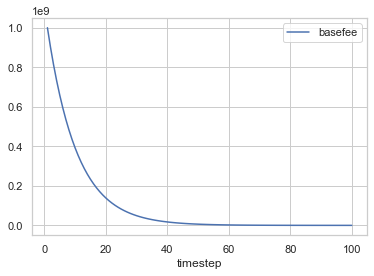Unsurprisingly, basefee decreases, in fact decreases quite fast to stabilise around just a few weis. We'll talk about the dynamics of basefee more when we dig into how the update is done.

### Refining the demand¶

Realistically though, our demand is not uniform at all. Some users will make a killing on Uniswap so would be happy to pay a high fee to have their transaction go through fast, while others are content to wait a bit longer should the entry price be too high. We'll refine the previous example a bit by adding some variability in the user transactions. We'll keep the gas used homogeneous, just to avoid solving complex set-packing problems.

In :
from random import randint

def update_demand_variable(params, step, sL, s, _input):
demand = {}

for i in range(100):
gas_premium = randint(1, 10) * (10 ** 9)
fee_cap = gas_premium + randint(1, 10) * (10 ** 9)
tx = Transaction(
gas_used = 21000,
fee_cap = fee_cap
)
demand[tx.tx_hash] = tx

return ("demand", demand)


Let's look into our demand a bit.

In :
def create_df_from_demand(demand):
fee_cap = pd.Series([tx.fee_cap for tx_hash, tx in demand.items()])

demand = {}

for i in range(100):
gas_premium = randint(1, 10) * (10 ** 9)
fee_cap = gas_premium + randint(1, 10) * (10 ** 9)
tx = Transaction(
gas_used = 21000,
fee_cap = fee_cap
)
demand[tx.tx_hash] = tx

demand_df = create_df_from_demand(demand)
demand_df.hist(bins = 10)

Out:
array([[<matplotlib.axes._subplots.AxesSubplot object at 0x1291164e0>,
<matplotlib.axes._subplots.AxesSubplot object at 0x1291a2710>]],
dtype=object)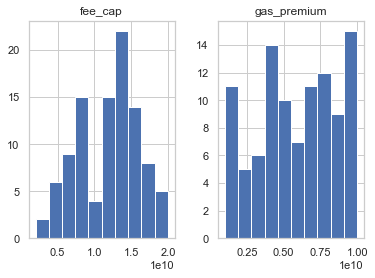The intuition behind these distributions is the following:

• gas_premium is obtained by throwing a ten-sided dice.
• To get fee_cap, we throw another ten-sided dice and add the result to the gas_premium we obtained.

We should find that gas_premium is somewhat uniformly distributed over 1 to 10 Gwei, while fee_cap looks more like a normal distribution (high chance of getting average values ~ 10-11, low chances of getting extreme values).

Since our average fee_cap will be close to 10 or 11 Gwei, let's start the basefee in our simulations to 10 Gwei. When a transaction sets its fee_cap lower than the prevailing basefee, it cannot be included in a block, since it is not willing to pay enough.

This time, we'll create a Block class to record the history of transactions getting in.

In :
class Block():
def __init__(self, txs):
self.txs = txs


We call a transaction valid if its feecap is higher than the prevailing basefee. Valid transactions are those that could potentially be included in a block (though may not be due to congestion, as we'll see later).

In :
def is_valid(tx, basefee):
return tx.fee_cap >= basefee


We redefine the block producer policy to return a block instead of simply the total gas used.

In :
def include_valid_txs(params, step, sL, s):
demand = s["demand"]
basefee = s["basefee"]
miner_gains = 0
txs_included = []

for tx_hash, tx in demand.items():
if not is_valid(tx, basefee):
continue

gas_price = min([basefee + tx.gas_premium, tx.fee_cap])
miner_gains += (gas_price - basefee) * tx.gas_used
txs_included += [tx]

assert miner_gains >= 0
return ({ "block": Block(txs = txs_included) })


This means we need to change our last state update.

In :
def update_basefee(params, step, sL, s, _input):
block = _input["block"]
basefee = s["basefee"]

gas_used = sum([tx.gas_used for tx in block.txs])
delta = gas_used - constants["TARGET_GAS_USED"]
new_basefee = basefee + basefee * delta // constants["TARGET_GAS_USED"] // constants["BASEFEE_MAX_CHANGE_DENOMINATOR"]

return ("basefee", new_basefee)


We'll also record the latest block in the state.

In :
def record_latest_block(params, step, sL, s, _input):
block = _input["block"]

return ("latest_block", block)


We'll assume for now that transactions which fail to get included just disappear, so a completely fresh new demand spawns for every block.

In :
%%capture

psub = [{
"policies": {},
"variables": {
"demand": update_demand_variable # step 1
}
}, {
"policies": {
"action": include_valid_txs # step 2
},
"variables": {
"basefee": update_basefee, # step 3
"latest_block": record_latest_block
}
}]

initial_conditions = {
"basefee": 10 * (10 ** 9),
"demand": {},
"latest_block": Block(txs=[])
}

simulation_parameters = {
'T': range(50),
'N': 1,
'M': {}
}

config = Configuration(initial_state=initial_conditions,
partial_state_update_blocks=psub,
sim_config=simulation_parameters
)

exec_mode = ExecutionMode()
exec_context = ExecutionContext(exec_mode.single_proc)
executor = Executor(exec_context, [config]) # Pass the configuration object inside an array
raw_result, tensor = executor.execute() # The execute() method returns a tuple; its first elements contains the raw results
df = pd.DataFrame(raw_result)


Let's check how the basefee evolves.

In :
df[df.substep == 1].plot('timestep', ['basefee'])

Out:
<matplotlib.axes._subplots.AxesSubplot at 0x129267550>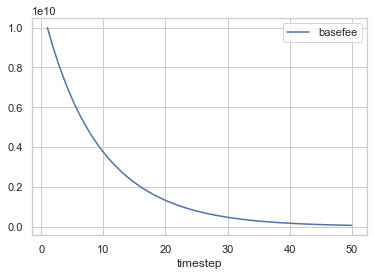As before, the basefee starts from high and progressively decreases, since blocks are not quite meeting their gas target. Let's see how many transactions get in each block.

In :
df["txs_per_block"] = df.latest_block.apply(
lambda block: len(block.txs)
)
df[(df.timestep > 1) & (df.substep == 1)].plot("timestep", "txs_per_block")

Out:
<matplotlib.axes._subplots.AxesSubplot at 0x115e052e8>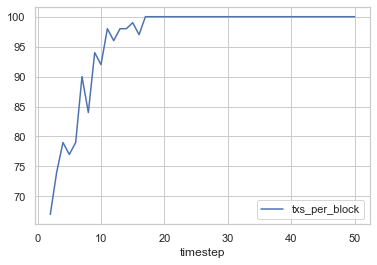The randomness is due to how we sample a new demand each step, but the trend is clear: as the basefee decreases, more and more transactions get in! We plot below the total premium collected by the producer and total basefee burned at each step.

In :
df["total_premium"] = df.apply(
lambda row: sum([tx.gas_premium for tx in row["latest_block"].txs]), axis = 1
)
df["total_basefee"] = df.apply(
lambda row: row["basefee"] * len(row["latest_block"].txs) + row["total_premium"], axis = 1
)
plot_data = df[df.substep == 2][["timestep", "total_basefee", "total_premium"]]

# Initialize the matplotlib figure
f, ax = plt.subplots(figsize=(15, 6))

sns.set_color_codes("pastel")
sns.barplot(x="timestep", y="total_basefee", data=plot_data,
label="Basefee", color="b")

sns.set_color_codes("muted")

ax.legend(ncol=2, loc="upper right", frameon=True)
ax.set(xlim=(-1, 50), ylabel="", xticklabels = [i if i % 5 == 0 else "" for i in range(1, 51)],
sns.despine(left=True, bottom=True)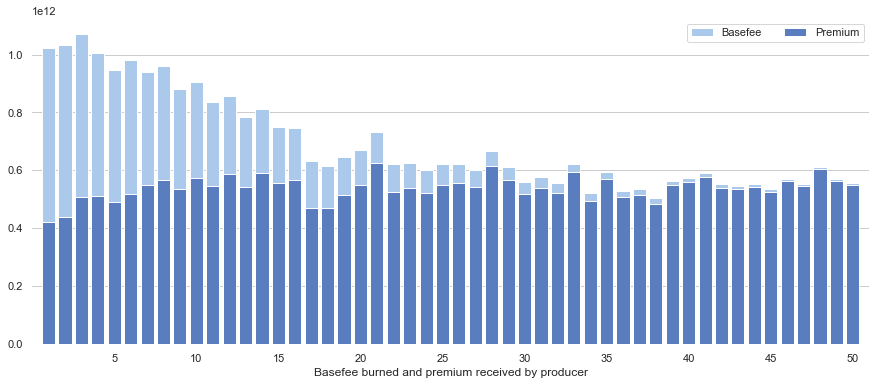### I'm overflow¶

So far we've only seen examples of a gas supply far in excess of the demand. Let's now see how the mechanism reacts when too many transactions are trying to get in.

There are tradeoffs for block producers when including transactions. The heavier the block (as in, the more transactions included), the greater the time for this block to be broadcasted over the P2P network of producers. This may not be such a problem in the eth2 instantiation of Proof-of-Stake, where your slot is "reserved" (see for instance our Beacon Runner) but in usual Proof-of-Work-backed consensus algorithms, being late to broadcast your block could mean that someone else was able to broadcast theirs, in which case your block won't be included in the canonical chain. This issue is mitigated by Ethereum's ommer mechanism in eth1.

We'll make an assumption here that block producers would allow their blocks to contain 20% over the gas target. That target being set currently at 10M in our constants, this means producers would allow blocks with 12M used gas. In practice, the size of the block (in bytes) is not in perfect relationship with the amount of gas used by the block, having more to do with the transaction size (in bytes). But since all our transactions are homogeneous, each using 21K gas, we can circumvent this issue. So blocks in the next simulation may feature up to 12M / 21K = 571 transactions (let's keep 570), while targeting 476 transactions (10M / 21K). Let's update our demand to reflect that, spawning 10,000 transactions each step.

In :
from random import uniform

def update_demand_variable(params, step, sL, s, _input):
demand = {}

for i in range(10000):
gas_premium = uniform(1, 11) * (10 ** 9)
fee_cap = gas_premium + uniform(1, 11) * (10 ** 9)
tx = Transaction(
gas_used = 21000,
fee_cap = fee_cap
)
demand[tx.tx_hash] = tx

return ("demand", demand)


Now block producers must choose which transactions to include. We'll rank transactions in order of their (decreasing) gas premium, since this is the bounty producers are getting out them.

In :
def include_valid_txs(params, step, sL, s):
demand = s["demand"]
basefee = s["basefee"]
timestep = s["timestep"]

sorted_valid_demand = sorted([tx for tx_hash, tx in demand.items() if is_valid(tx, basefee)], key = lambda tx: -tx.gas_premium)
included_transactions = sorted_valid_demand[0:570]

return ({ "block": Block(txs = included_transactions) })


Simulate it...

In :
%%capture
psub = [{
"policies": {},
"variables": {
"demand": update_demand_variable # step 1
}
}, {
"policies": {
"action": include_valid_txs # step 2
},
"variables": {
"basefee": update_basefee, # step 3
"latest_block": record_latest_block # bonus step
}
}]

initial_conditions = {
"basefee": 10 * (10 ** 9),
"demand": {},
"latest_block": Block(txs=[])
}

simulation_parameters = {
'T': range(50),
'N': 1,
'M': {}
}

config = Configuration(initial_state=initial_conditions,
partial_state_update_blocks=psub,
sim_config=simulation_parameters
)

exec_mode = ExecutionMode()
exec_context = ExecutionContext(exec_mode.single_proc)
executor = Executor(exec_context, [config]) # Pass the configuration object inside an array
raw_result, tensor = executor.execute() # The execute() method returns a tuple; its first elements contains the raw results
df = pd.DataFrame(raw_result)


Let's plot the basefee.

In :
df[df.substep == 1].plot('timestep', ['basefee'])

Out:
<matplotlib.axes._subplots.AxesSubplot at 0x115b08390>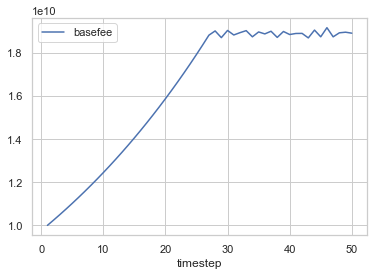Is that Mt. Fuji? Basefee stabilises around 19 Gwei, with oscillations due to the randomness of our demand. We can see why by inspecting how many valid transactions don't make it in the block.

Since we generate 10,000 transactions for each block with uniform distribution between 1 and 11 for gas_premium, we should expect (in the probabilistic sense) that our 476 most juiciest transactions (measured by gas_premium) will have a gas_premium of at least 10.524 Gwei. On the other hand, block producers must make sure that the fee_cap is enough to cover the basefee, so they can't be too picky either and might have to choose transactions that have a high enough fee_cap, regardless of their gas_premium. Over time then, the average premium decreases, as seen in the following plot:

In :
df["average_premium"] = df.latest_block.apply(
lambda block: 0 if len(block.txs) == 0 else float(sum([tx.gas_premium for tx in block.txs])) / len(block.txs)
)
df[(df.timestep > 1) & (df.substep == 1)].plot("timestep", "average_premium")

Out:
<matplotlib.axes._subplots.AxesSubplot at 0x1292db940>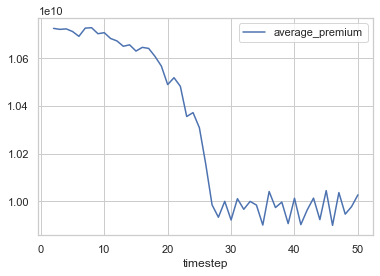To recover a block size at the desired target, the basefee increases, pricing out more and more transactions. Let's check the percentage of valid transactions (i.e., tx.fee_cap > basefee) which are not included in the block.

In :
df["previous_basefee"] = df.basefee.shift(1)
df["txs_per_block"] = df.latest_block.apply(
lambda block: len(block.txs)
)
df["valid_transactions"] = df.apply(
lambda row: len([tx for tx_hash, tx in row.demand.items() if is_valid(tx, row.previous_basefee)]), axis=1
)
df["fraction_priced_out"] = df.apply(
lambda row: 0 if row.valid_transactions == 0 else (1 - float(row.txs_per_block) / float(row.valid_transactions)) * 100, axis=1
)

In :
df[(df.timestep > 1) & (df.substep == 2)].plot("timestep", "fraction_priced_out")

Out:
<matplotlib.axes._subplots.AxesSubplot at 0x12928bef0>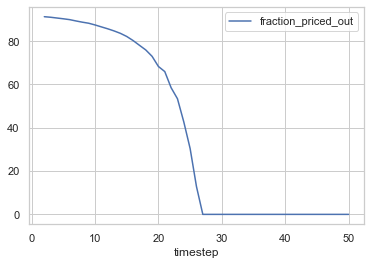That number goes to zero pretty fast, meaning that previously valid (but due to size constraints, unincluded) transactions become invalid as the basefee rises. How about the gas used by the blocks?

In :
df["gas_per_block"] = df.latest_block.apply(
lambda block: sum([tx.gas_used for tx in block.txs])
)
df[(df.timestep > 1) & (df.substep == 2)].plot("timestep", "gas_per_block")

Out:
<matplotlib.axes._subplots.AxesSubplot at 0x139618198>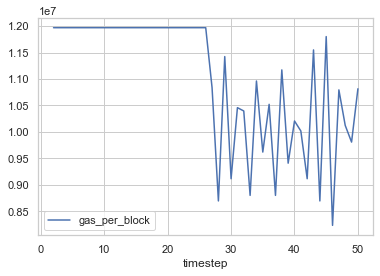We get close to 10M gas per block (on average, with some variability due to random effects) around the 27th block, which is the target we had set. Victory!

Let's plot again the total basefee burned and premium collected.

In :
df["total_premium"] = df.apply(
lambda row: sum([tx.gas_premium for tx in row["latest_block"].txs]), axis = 1
)
df["total_basefee"] = df.apply(
lambda row: row["basefee"] * len(row["latest_block"].txs) + row["total_premium"], axis = 1
)
plot_data = df[df.substep == 2][["timestep", "total_basefee", "total_premium"]]

sns.set(style="whitegrid")

# Initialize the matplotlib figure
f, ax = plt.subplots(figsize=(15, 6))

sns.set_color_codes("pastel")
sns.barplot(x="timestep", y="total_basefee", data=plot_data,
label="Basefee", color="b")

sns.set_color_codes("muted")

ax.legend(ncol=2, loc="upper right", frameon=True)
ax.set(xlim=(-1, 50), ylabel="", xticklabels = [i if i % 5 == 0 else "" for i in range(1, 51)],
sns.despine(left=True, bottom=True)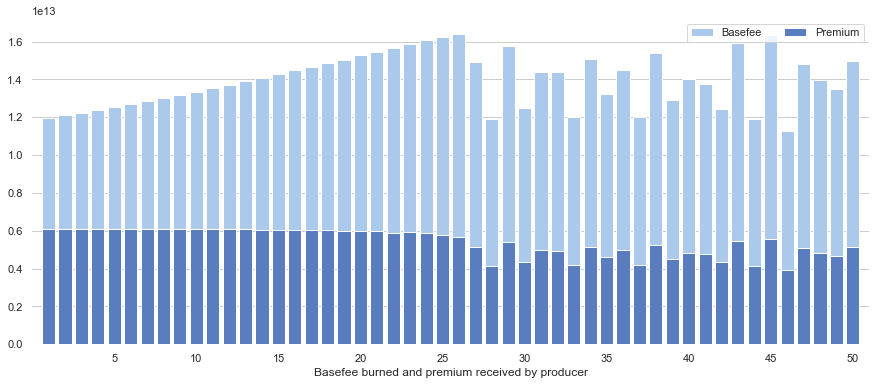### Bottomless pit¶

When a full block is produced, transactions that were not included do not suddenly disappear. Instead, the mempool fills up with unincluded transactions. It is time to add a bit of memory to our leaky mempool.

Now we might want to be a bit careful since two things can happen:

• All transactions are included always. That's not very interesting. We would like to observe the effects over time of congestion, so we should once in a while have a greater demand than we have supply.
• New transactions always exceed supply. That's no good either, and our model would quickly blow up. Unless we introduce some kind of staling, our mempool would soon be overloaded.

We try to settle for some middle ground, where in some steps we have more transactions coming in than a block can fit, and in others the demand is not too consequential, allowing blocks to catch up with older transactions.

We'll keep again transactions at a fixed size of 21,000 gas, but will introduce a new object to our simulation, the scenario. It is a simple array that contains at index $t$ the number of transactions coming in at time $t$ of our simulation. Since we are aiming for a block size of 10M gas, and we have seen that we can fit 476 transactions asking for 21,000 gas in one block, the values in our scenario will oscillate around 476 --- sometimes more, sometimes less.

In :
from math import sin, pi

timesteps = 300

def generate_oscillating_scenario(timesteps):
return [int(400 + 200 * sin(i * pi / 16.0)) for i in range(timesteps)]

tx_scenario = generate_oscillating_scenario(timesteps)
pd.DataFrame({ "txs": tx_scenario, "producer_limit": 570, "target_gas": 476 }).plot()

Out:
<matplotlib.axes._subplots.AxesSubplot at 0x129302128>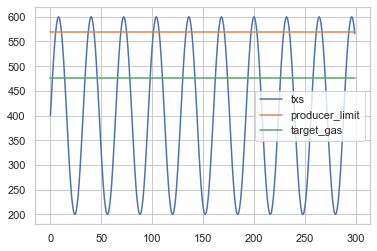(We'll also drop cadCAD here and run the simulations with our own code. The size of our demand object can grow big and cadCAD deep copies this state by default, which is not necessary here but slows the simulation down quite a bit. Finding a workaround is on my bucket list! In the meantime, you can check in this notebook how to run the remaining parts with cadCAD (this is how I originally wrote them), you'll find a much cleaner and more elegant code than the following.)

In :
def update_demand_scenario(timestep, demand, tx_scenario):
for i in range(tx_scenario[timestep]):
gas_premium = uniform(1, 11) * (10 ** 9)
fee_cap = gas_premium + uniform(1, 11) * (10 ** 9)
tx = Transaction(
gas_used = 21000,
fee_cap = fee_cap
)
demand[tx.tx_hash] = tx

return demand

In :
def include_valid_txs(demand, basefee):
sorted_valid_demand = sorted([tx for tx_hash, tx in demand.items() if is_valid(tx, basefee)], key = lambda tx: -tx.gas_premium)
included_transactions = sorted_valid_demand[0:570]

return Block(txs = included_transactions)

In :
def update_basefee(block, basefee):
gas_used = sum([tx.gas_used for tx in block.txs])
delta = gas_used - constants["TARGET_GAS_USED"]
new_basefee = basefee + basefee * delta // constants["TARGET_GAS_USED"] // constants["BASEFEE_MAX_CHANGE_DENOMINATOR"]

return new_basefee

In :
def remove_included_txs(demand, latest_block):
for tx in latest_block.txs:
del(demand[tx.tx_hash])

return demand

In :
%%capture
T = timesteps

basefee = 5 * (10 ** 9)
demand = {}
latest_block = Block(txs = [])
rows = [{ "timestep": 0, "basefee": basefee, "demand": demand, "latest_block": latest_block }]

for timestep in range(T):
demand = update_demand_scenario(timestep, demand, tx_scenario)
latest_block = include_valid_txs(demand, basefee)
basefee = update_basefee(latest_block, basefee)
demand = remove_included_txs(demand, latest_block)
rows += [{ "timestep": timestep, "basefee": basefee, "demand": demand.copy(), "latest_block": latest_block }]

df = pd.DataFrame(rows)

In :
df.plot("timestep", "basefee")

Out:
<matplotlib.axes._subplots.AxesSubplot at 0x1394b9908>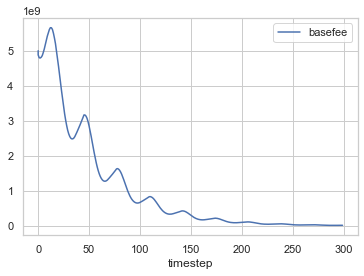In :
df["mempool_size"] = df.demand.apply(
lambda demand: len(demand)
)
df.plot("timestep", "mempool_size")

Out:
<matplotlib.axes._subplots.AxesSubplot at 0x13b6f9d68>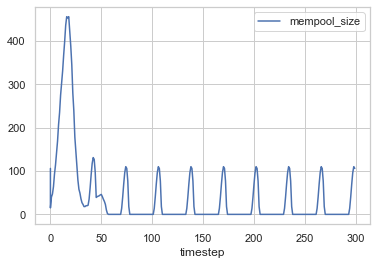In :
df["included_txs"] = df.latest_block.apply(
lambda block: len(block.txs)
)
df[df.timestep > 0].plot("timestep", "included_txs")

Out:
<matplotlib.axes._subplots.AxesSubplot at 0x13b7970b8>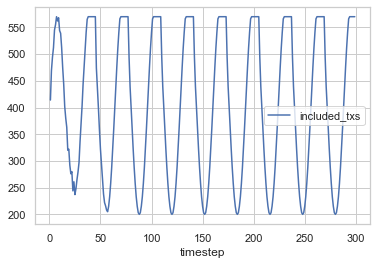### Spikes¶

Tron 2: Legacy decides to ICO on Ethereum and everyone wants in! Transactions are spiking to 10,000 of them between two blocks, while we know our blocks accommodate only about 570 max at a time...

In :
from math import pow, exp

timesteps = 300

def generate_spike_scenario(timesteps):
spikey_boi = timesteps // 8
return [int(10000 * exp(-pow(i - spikey_boi, 2)/16.0)) for i in range(timesteps)]

tx_scenario = generate_spike_scenario(timesteps)
pd.DataFrame({ "txs": tx_scenario, "producer_limit": 570, "target_gas": 476 }).plot()

Out:
<matplotlib.axes._subplots.AxesSubplot at 0x13b7fb5c0>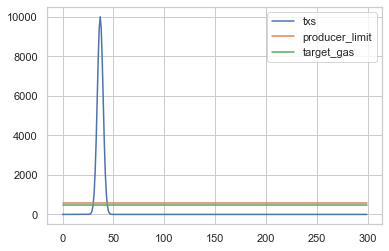In :
%%capture
T = timesteps

basefee = 5 * (10 ** 9)
demand = {}
latest_block = Block(txs = [])
rows = [{ "timestep": 0, "basefee": basefee, "demand": demand, "latest_block": latest_block }]

for timestep in range(T):
demand = update_demand_scenario(timestep, demand, tx_scenario)
latest_block = include_valid_txs(demand, basefee)
basefee = update_basefee(latest_block, basefee)
demand = remove_included_txs(demand, latest_block)
rows += [{ "timestep": timestep, "basefee": basefee, "demand": demand.copy(), "latest_block": latest_block }]

df = pd.DataFrame(rows)


We start from a high basefee but it's all quiet at first so basefee drops. Around timestep 30 though boom, transactions roll in and the basefee nudges upwards, reaching its apex around timestep 150, once all leftover transactions from the spike were processed.

In :
df.plot("timestep", "basefee")

Out:
<matplotlib.axes._subplots.AxesSubplot at 0x13b96b5c0>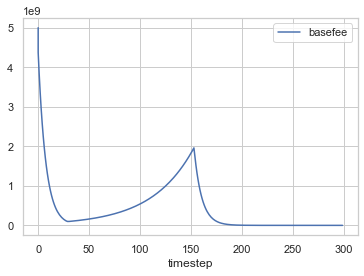We clearly see the mempool size spiking in concert with the demand spike, and linearly emptying until the 150th timestep.

In :
df["mempool_size"] = df.demand.apply(
lambda demand: len(demand)
)
df.plot("timestep", "mempool_size")

Out:
<matplotlib.axes._subplots.AxesSubplot at 0x13b96b7b8>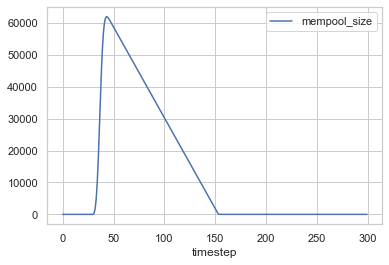Blocks are either empty or full at this point:

In :
df["included_txs"] = df.latest_block.apply(
lambda block: len(block.txs)
)
df.plot("timestep", "included_txs")

Out:
<matplotlib.axes._subplots.AxesSubplot at 0x14747eb00>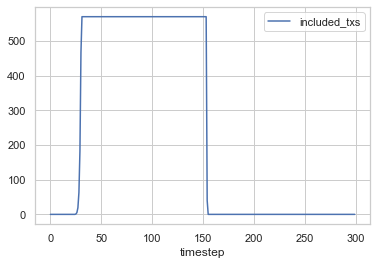Some pretty Diracs, triangle and square waves... Plug in a few synthesisers to this notebook and you'll get a Tangerine Dream album right there.

## A more realistic demand¶

Let's summarise what we have done so far. We made some pretty strong assumptions on the demand, looking at an exogenous demand (i.e., we set it outside of our model, as a parameter). For now, this demand is really simple: people only want to use the chain to do a transfer, which uses 21,000 units of gas. If they are not included at the time their transaction is sent, they hold on until inclusion, i.e., they have really weak to inexistent time preferences. Given the demand that they observe, block producers select which candidate transactions to include in their blocks.

This doesn't tell us much about our mechanism though. We would like instead to study how a more realistic demand for gas will change the behaviour of the basefee and the transaction fee market. Users may set their parameters based on their observations of the market for instance, deciding to put a large tip if they observe high competition that wasn't yet matched by the basefee.

To instantiate a reasonable model of the demand, it is useful to glean some insights into how the demand behaved historically. Of course, we do not directly have access to this historical demand --- to do so, we would have needed to monitor every change of the mempool over time. What we do have access to though is the history of included transactions in blocks, including the gas price and total fee they paid to be included.

Since the historical series of fees was determined by the first-price auction mechanism, we cannot completely expect that the realised demand under a new mechanism will be the same. Maybe our EIP 1559 fee market is really good at coping with congestion and doesn't incent users to be strategic about their bidding, the way they were under the auction mechamism, leading to big price spikes during particularly juicy ICOs or caring for furry beings. In that case, the realised demand observed with the auction won't be a very good prediction for the demand under EIP 1559. We could look into revealed preferences, the science of inferring what people want from what they do, to help us pin down the main determinants and instead draw a better model of the demand.

This is all a bit too long, so we'll leave that chunk for a future notebook. Thanks for reading and stay safe ❤️

Acknowledgements: James for prompting this research in the first place; Vitalik for helpful comments on the draft.